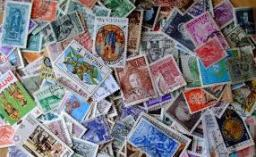# Three-digit 4882

There were 301 postage stamps in the desk drawer. They were stamps for 2, 3, and 5 crowns. The number of each species can be written as a three-digit number. The total price of all stamps is CZK 1,003. How many stamps were there?

a =  100
b =  101
c =  100

### Step-by-step explanation:Did you find an error or inaccuracy? Feel free to write us. Thank you!

Tips for related online calculators
Do you have a system of equations and looking for calculator system of linear equations?